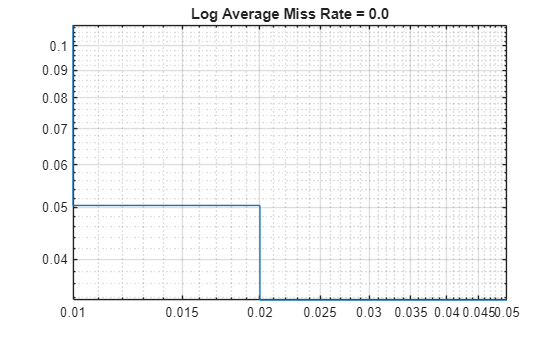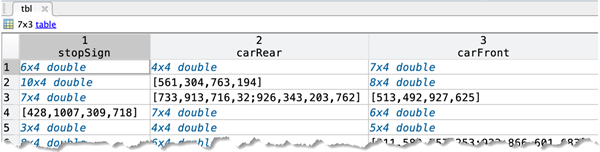# evaluateDetectionMissRate

Evaluate miss rate metric for object detection

## Syntax

``logAverageMissRate = evaluateDetectionMissRate(detectionResults,groundTruthData)``
``````[logAverageMissRate,fppi,missRate] = evaluateDetectionMissRate(___)``````
``[___] = evaluateDetectionMissRate(___,threshold)``

## Description

example

````logAverageMissRate = evaluateDetectionMissRate(detectionResults,groundTruthData)` returns the log-average miss rate of the `detectionResults` compared to `groundTruthData`, which is used to measure the performance of the object detector. For a multiclass detector, the log-average miss rate is a vector of scores for each object class in the order specified by `groundTruthData`.```

example

``````[logAverageMissRate,fppi,missRate] = evaluateDetectionMissRate(___)``` returns data points for plotting the log miss rate–false positives per image (FPPI) curve, using input arguments from the previous syntax.```
````[___] = evaluateDetectionMissRate(___,threshold)` specifies the overlap threshold for assigning a detection to a ground truth box.```

## Examples

collapse all

This example shows how to evaluate miss rate of a pretrained YOLO v2 object detector.

Load the Vehicle Ground Truth Data

Load a table containing the vehicle training data. The first column contains the training images, the remaining columns contain the labeled bounding boxes.

```data = load('vehicleTrainingData.mat'); trainingData = data.vehicleTrainingData(1:100,:);```

Add fullpath to the local vehicle data folder.

```dataDir = fullfile(toolboxdir('vision'), 'visiondata'); trainingData.imageFilename = fullfile(dataDir, trainingData.imageFilename);```

Create an imageDatastore using the files from the table.

`imds = imageDatastore(trainingData.imageFilename);`

Create a boxLabelDatastore using the label columns from the table.

`blds = boxLabelDatastore(trainingData(:,2:end));`

Load the detector containing the layerGraph for trainining.

```vehicleDetector = load('yolov2VehicleDetector.mat'); detector = vehicleDetector.detector;```

Evaluate and Plot the Miss Rate Results

Run the detector with imageDatastore.

`results = detect(detector, imds);`

Evaluate the results against the ground truth data.

`[am, fppi, missRate] = evaluateDetectionMissRate(results, blds);`

Plot the log-miss-rate/FPPI curve.

```figure; loglog(fppi, missRate); grid on title(sprintf('Log Average Miss Rate = %.1f', am))``````load('stopSignsAndCars.mat') stopSigns = stopSignsAndCars(:, 1:2); stopSigns.imageFilename = fullfile(toolboxdir('vision'),'visiondata', ... stopSigns.imageFilename);```

Train an ACF based detector.

`detector = trainACFObjectDetector(stopSigns,'NegativeSamplesFactor',2);`
```ACF Object Detector Training The training will take 4 stages. The model size is 34x31. Sample positive examples(~100% Completed) Compute approximation coefficients...Completed. Compute aggregated channel features...Completed. -------------------------------------------- Stage 1: Sample negative examples(~100% Completed) Compute aggregated channel features...Completed. Train classifier with 42 positive examples and 84 negative examples...Completed. The trained classifier has 19 weak learners. -------------------------------------------- Stage 2: Sample negative examples(~100% Completed) Found 84 new negative examples for training. Compute aggregated channel features...Completed. Train classifier with 42 positive examples and 84 negative examples...Completed. The trained classifier has 20 weak learners. -------------------------------------------- Stage 3: Sample negative examples(~100% Completed) Found 84 new negative examples for training. Compute aggregated channel features...Completed. Train classifier with 42 positive examples and 84 negative examples...Completed. The trained classifier has 54 weak learners. -------------------------------------------- Stage 4: Sample negative examples(~100% Completed) Found 84 new negative examples for training. Compute aggregated channel features...Completed. Train classifier with 42 positive examples and 84 negative examples...Completed. The trained classifier has 61 weak learners. -------------------------------------------- ACF object detector training is completed. Elapsed time is 16.0058 seconds. ```

Create a struct array to store the results.

```numImages = height(stopSigns); results(numImages) = struct('Boxes', [], 'Scores', []);```

Run the detector on the training images.

```for i = 1 : numImages I = imread(stopSigns.imageFilename{i}); [bboxes, scores] = detect(detector, I); results(i).Boxes = bboxes; results(i).Scores = scores; end results = struct2table(results);```

Evaluate the results against the ground truth data.

`[am, fppi, missRate] = evaluateDetectionMissRate(results, stopSigns(:, 2));`

Plot log-miss-rate/FPPI curve.

```figure loglog(fppi, missRate); grid on title(sprintf('log Average Miss Rate = %.1f', am))```## Input Arguments

collapse all

Object locations and scores, specified as a two-column table containing the bounding boxes and scores for each detected object. For multiclass detection, a third column contains the predicted label for each detection. The bounding boxes must be stored in an M-by-4 cell array. The scores must be stored in an M-by-1 cell array, and the labels must be stored as a categorical vector.

When detecting objects, you can create the detection results table by using `imageDatastore`.

``` ds = imageDatastore(stopSigns.imageFilename); detectionResults = detect(detector,ds);```

Data Types: `table`

Labeled ground truth, specified as a datastore or a table.

Each bounding box must be in the format [x y width height].

• Datastore — A datastore whose `read` and `readall` functions return a cell array or a table with at least two columns of bounding box and labels cell vectors. The bounding boxes must be in a cell array of M-by-4 matrices in the format [x,y,width,height]. The datastore's `read` and `readall` functions must return one of the formats:

• Table — One or more columns. All columns contain bounding boxes. Each column must be a cell vector that contains M-by-4 matrices that represent a single object class, such as stopSign, carRear, or carFront . The columns contain 4-element double arrays of M bounding boxes in the format [x,y,width,height]. The format specifies the upper-left corner location and size of the bounding box in the corresponding image.Overlap threshold for a detection assigned to a ground truth box, specified as a numeric scalar. The overlap ratio is computed as the intersection over union.

## Output Arguments

collapse all

Log-average miss rate metric, returned as either a numeric scalar or vector. For a multiclass detector, the log-average miss rate is returned as a vector of values that correspond to the data points for each class.

False positives per image, returned as either a vector of numeric scalars or as a cell array. For a multiclass detector, the FPPI and log miss rate are cell arrays, where each cell contains the data points for each object class.

Log miss rate, returned as either a vector of numeric scalars or as a cell array. For a multiclass detector, the FPPI and log miss rate are cell arrays, where each cell contains the data points for each object class.

## Version History

Introduced in R2017a